# Physics Assignment Help With Combination of Resistors

## 11.6 Combination of Resistors

### 11.6.1 Resistors in Series

Two resistors are said to be in series if the same current passes through both resistors. For example, in the circuit shown, any current coming from the battery must pass through both resistors – the current has no place else to go.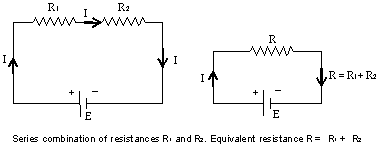At every instant of time all circuit elements connected in a series combination carry the same current. The equivalent resistance of N resistors connected in series is given by

R = R1 + R2 + ….+ RN =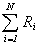The equivalent resistance of a series combination of resistances is always larger than any of the individual resistances.

### 11.6.2 Resistors in Parallel

Two resistors are said to be in parallel combination if the current I splits into two currents I1 and I2 such that I = I1 + I2 but the voltage drop across each resistor is the same.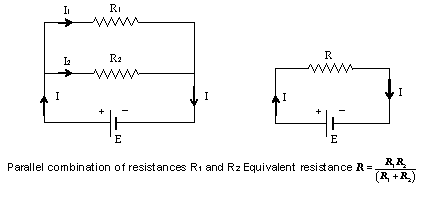For N resistors are connected in a parallel combination, the equivalent resistance is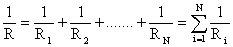The total resistance of any parallel combination of resistors must be less than any one of the individual resistors.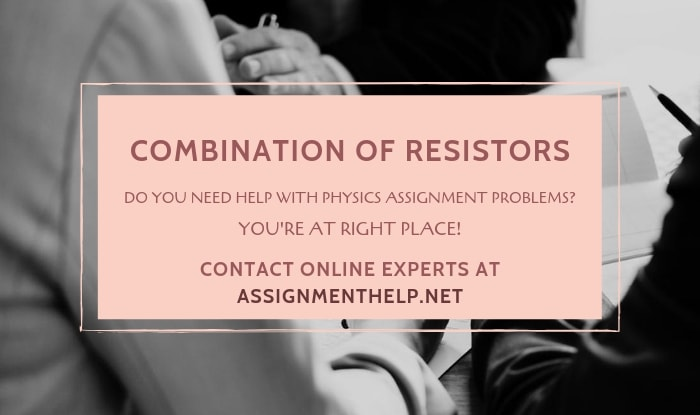### Email Based Assignment Help in Combination of Resistors

We are the leading online Assignment Help provider. Find answers to all of your doubts regarding the Combination of Resistors. Assignmenthelp.net provide homework, Assignment Help to the school, college or university level students. Our expert online tutors are available to help you in Combination of Resistors. Our service is focused on: time delivery, superior quality, creativity and originality.